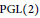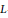Hostname: page-component-594f858ff7-wfvfs Total loading time: 0 Render date: 2023-06-10T07:53:00.391Z Has data issue: false Feature Flags: { "corePageComponentGetUserInfoFromSharedSession": true, "coreDisableEcommerce": false, "corePageComponentUseShareaholicInsteadOfAddThis": true, "coreDisableSocialShare": false, "useRatesEcommerce": true } hasContentIssue false

# Existence of Hilbert Cusp Forms with Non-vanishing L-values

Published online by Cambridge University Press:  20 November 2018

## Abstract

HTML view is not available for this content. However, as you have access to this content, a full PDF is available via the ‘Save PDF’ action button.

We develop a derivative version of the relative trace formula on$\text{PGL}\left( 2 \right)$ studied in our previous work, and derive an asymptotic formula of an average of central values (derivatives) of automorphic$L$-functions for Hilbert cusp forms. As an application, we prove the existence of Hilbert cusp forms with non-vanishing central values (derivatives) such that the absolute degrees of their Hecke fields are arbitrarily large.

## Keywords

Type
Research Article
Information
Canadian Journal of Mathematics , 01 August 2016 , pp. 908 - 960

## References

 Gross, B. H., and Zagier, D. B., Heegner points and derivatives ofL-series. Inventiones Math. 84(1986), 225320. http://dx.doi.org/10.1007/BF01388809 Google Scholar
 Jacquet, H., and Chen, N., Positivity of quadratic base change L-functions. Bull. Soc. Math.France 129(2001), no. 1, 3390.Google Scholar
 Jacquet, H., and Langlands, L. P., Automorphic forms onGL(2). Lecture Notes in Mathematics, 114, Springer-Verlag, Berlin, 1970.CrossRefGoogle Scholar
 Magnus, W., Oberhettinger, E., and Soni, R., Formulas and theorems for the special functions of mathematical physics. In: Grundlehren der mathematischen Wissenschafter, 52, 3rd edition. Springer-Verlag, NewYork, 1966.CrossRefGoogle Scholar
 Raghuram, A. and Tanabe, N., Notes of the arithmetic ofHilbert modular forms. J. Ramanujan Math. Soc. 26(2011), no. 3, 261319.Google Scholar
 Royer, E., Facteurs Q-simples de Jo (N) de grande dimension et de grand rang. Bull. Soc. Math.France 128(2000), no. 2, 219248.Google Scholar
 Serre, J.P., Répartition asymptotique des valeurs propres de l'operateur de Hecke Tp. J. Amer. Math.Soc. 10(1997), no. 1, 75102.http://dx.doi.org/10.1090/S0894-0347-97-00220-8 Google Scholar
 Shimura, G., The special values of the zêta functions associated with Hilbert modular forms. Duke Math. J. 45(1978), no.3, 637679.http://dx.doi.org/10.1215/S0012-7094-78-04529-5 Google Scholar
 Sugiyama, S., Regularized periods of automorphic forms on GL(2). Tohoku Math. J. 65(2013), no. 3, 73409.http://dx.doi.org/10.2748/tmj71378991022 Google Scholar
 Sugiyama, S., Asymptotic behaviors of means of central values of automorphic L-functions for GL(2). J.Number Theory, 156(2015), 195246.http://dx.doi.Org/10.1016/j.jnt.2O15.04.003 Google Scholar
 Sugiyama, S., and Tsuzuki, M., Relative trace formulas and subconvexity estimates of L-functions for Hilbert modular forms (preprint 2013). arxiv:http://arxiv.org/abs/1305.2261 Google Scholar
 Tsuzuki, M., Spectral means of central values of automorphic L-functions for GL(2). Mem. Amer.Math. Soc. 235(1110), 2015.http://dx.doi.Org/10.1090/memo/1110 Google Scholar
 Waldspurger, J. L., Sur les valeurs de certaines fonctions L automorphes en leur centre de symmétrie. Compositio Math. 54(1985), 173242.Google Scholar
 Yuan, X., Zhang, S. W., and Zhang, W., The Gross-Zagier formula on Shimura curves. Annals of Mathematics Studies, 184, Princeton University Press, Princeton, NJ, 2013.Google Scholar
 Zhang, S. W., Heights of Heegner cycles and derivatives ofL-series. Invent. Math. 130(1997), 99152.http://dx.doi.org/10.1007/s002220050179 Google Scholar
 Zhang, S. W., Gross-Zagier formula forGL(2). Asian J. Math. 5(2001), 183290.http://dx.doi.org/10.4310/AJM.2001.v5.n2.a1 Google Scholar
 Zhang, S. W., Gross-Zagier formula forGL(2). II. In: Heegner points and Rankin L-series. Math. Sci.Res. Inst. Publ. 49, Cambridge University Press, Cambridge, 2004, pp. 191214.http://dx.doi.ore/10.1017/CBO9780511756375.008 Google Scholar